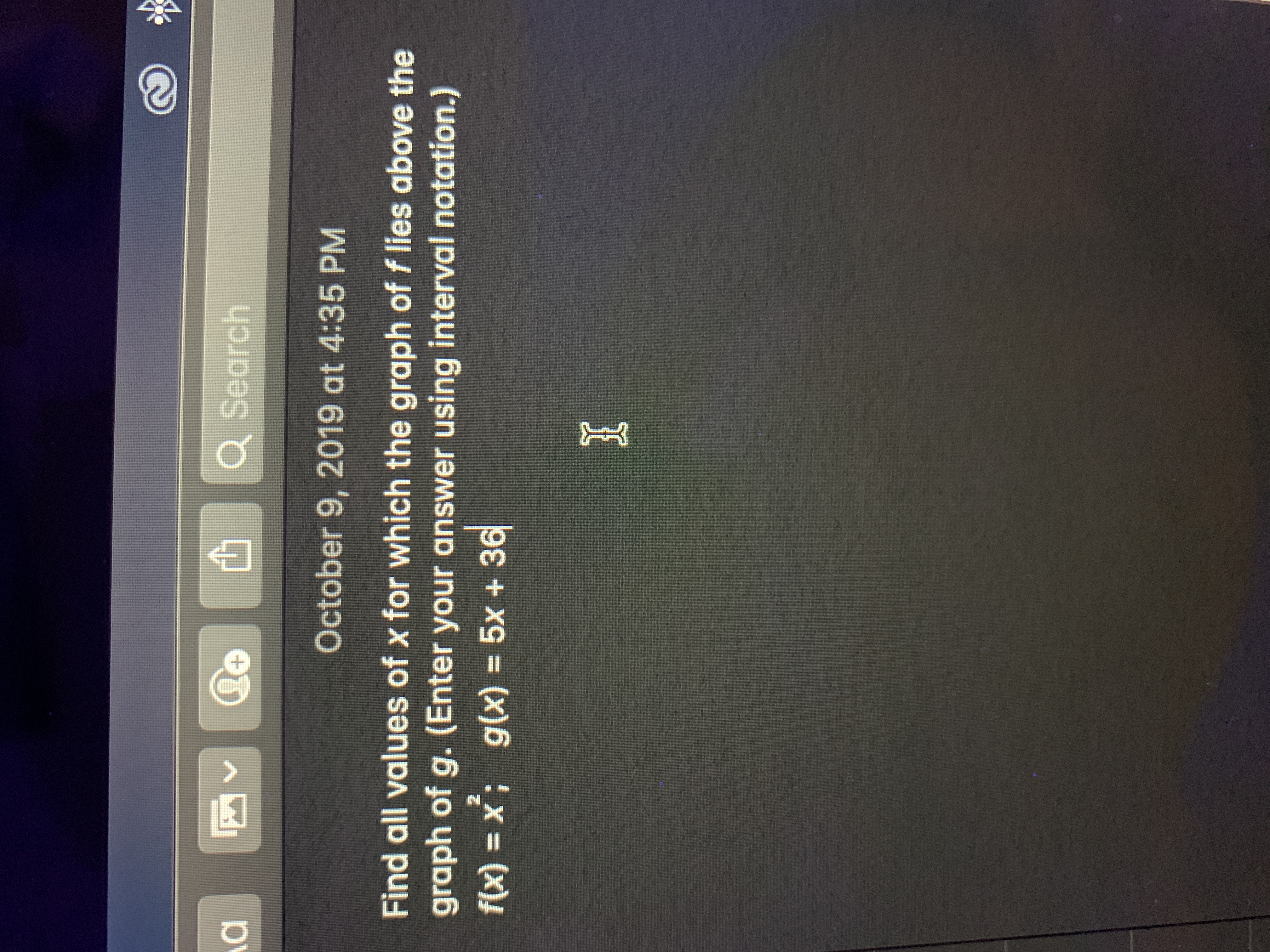# Q SearchOctober 9, 2019 at 4:35 PMFind all values of x for which the graph of f lies above thegraph of g. (Enter your answer using interval notation.)f(x) x; g(x) = 5x + 362

Question
3 viewshelp_outlineImage TranscriptioncloseQ Search October 9, 2019 at 4:35 PM Find all values of x for which the graph of f lies above the graph of g. (Enter your answer using interval notation.) f(x) x; g(x) = 5x + 36 2 fullscreen
check_circle

Step 1

It is given that, f (x) = x2 and f (x) = 5x+36.

Here, the graph of f lies above the graph of g.

That is, f (...

### Want to see the full answer?

See Solution

#### Want to see this answer and more?

Solutions are written by subject experts who are available 24/7. Questions are typically answered within 1 hour.*

See Solution
*Response times may vary by subject and question.
Tagged in

### Other